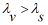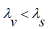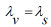Wave Mechanics homework Help at TutorEye

## Wave Mechanics:

Wave Mechanics is the branch of quantum physics, which deals in the behaviour of particles as waves. It was originally developed by the Austrian physicist Erwin Schrodinger. It describes any elementary particles in terms of their wavelike properties. The theory of wave mechanics interprets the state and motion of subatomic or small particles by the properties of waves.

## Wave MechanicsSample Questions:

Question 1: Which of the following statements is false?

a) Sound is a form of energy                 b) Sound propagates in the form of waves

c) Sound travels in straight lines           d) Sound travel faster in vacuum than air

Answer: d) Sound travel faster in vacuum than air

Explanation: Since, air is a rarer medium than

Question 2: The minimum audible wavelength at room temperature is

a) 10 mm             b) 30 mm

c) 20 mm             d) 40 mm

Explanation: Maximum audible frequency at room temperature = 20,000 Hz

Question 3: The distance between two consecutive crests produced in a string is 10cm. If three waves pass through a point per second, the velocity of the wave is

a) 30 cm/s           b) 20 m/s

c) 10 cm/s           d) 40 m/s

Explanation: Given,

Question 4: The wavelength of light in visible part and for sound are related as

a)b)c)d) None of these

Answer: b)Explanation: As sound travels millions of times slower

Question 5: In a sinusoidal wave, the time required for a point to travel from maximum displacement to zero displacement is 0.5 seconds. What is the frequency of the wave?

a) 0.1 Hz         b) 0.5 Hz

c) 0.2 Hz         d) 0.2 Hz

Explanation: Time required by a point to travel from maximum displacement to zero displacement is

Question 6: In air, sound travels 5 km in 10 sec and in another medium, it travels 2 km in 3 sec.The ratio of the wavelength is

a) 1:2          b) 3:4

c) 4:3          d) 2:1

Explanation:  Given,

Question 7: The temperature at which velocity of sound is twice that of at zero degree is

a) 500 K                b) 500° C

c) 819° C              c) 819 K

Explanation: Since,

Question 8: Velocity of sound is maximum in

a) air              b) vacuum

c) steel            d) water

Explanation: Since the velocity of sound in

Question 9: The speed of a wave in a medium is 1000 m/s. If 200 waves pass from a point of the medium in 1 minute. The wavelength is

a) 300 m              b) 100 m

c) 200 m              d) 400 m

Explanation: Given,

Question 10: Velocity of sound in air is

a) Directly proportional to temperature             b) Directly proportional to pressure

c) Directly proportional to wavelength              d) Independent of the pressure of air

Answer: d) Independent of the pressure of air

Explanation: As P changes ρ also changes, the ratio of P